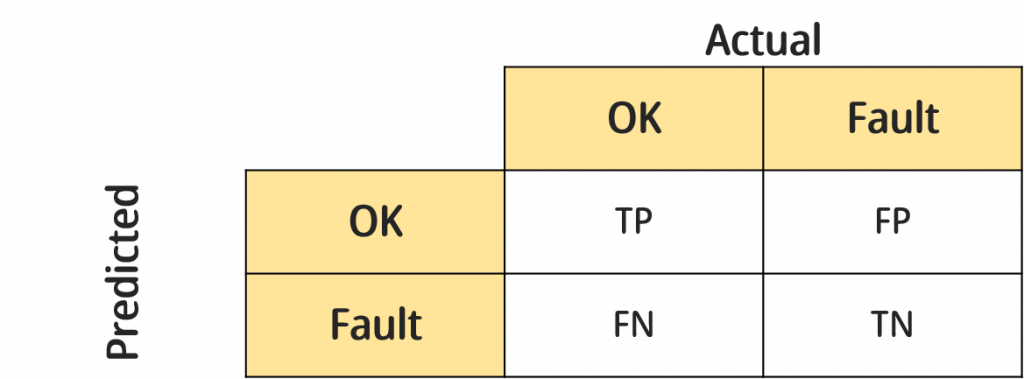#DAY 15
0
AI & Data

## 一、回歸問題評估方式-MSE、MAE

### MAE(絕對平均誤差)

``````#MSE
from sklearn.metrics import mean_squared_error
y_true = [3, -0.5, 2, 7] #真實值
y_pred = [2.5, 0.0, 2, 8] #預測值
mean_squared_error(y_true, y_pred)
``````
``````#MAE
from sklearn.metrics import mean_absolute_error
y_true = [3, -0.5, 2, 7] #真實值
y_pred = [2.5, 0.0, 2, 8] #預測值
mean_absolute_error(y_true, y_pred)
``````

## 二、分類問題評估方式-混淆矩陣(Confution Matrix)

(備註：矩陣會隨預測的標籤數增加，若標籤為n個，矩陣大小就為n*n)### 混淆矩陣TP、FP、FN、TN意義

▲True Positive(TP)：實際狀態為OK且預測狀態也為OK。

▲False Positive(FP)：實際狀態為Fault但預測狀態為OK。

▲False Negative(FN)：實際狀態為OK但預測狀態為Fault。

▲True Negative(TN)：實際狀態為Fault且預測狀態為Fault。

False Positive(FP)又稱偽陽性，明明產品是壞掉的，我們卻沒有檢驗出來，反而說它是好的，這在統計學的假設檢定中又稱為型一錯誤，品管中我們稱之為消費者風險(因為消費者會拿到壞的產品)。

False Negative(FN)又稱偽陰性，產品是正常的，我們卻說他是壞的，統計學的假設檢定中稱為型二錯誤，品管中稱為生產者風險(因為把好的產品挑出來浪費掉了)。

### 延伸指標

Accuracy：最單純的準確率，預測正確的比例。

Precision：所有負樣本中，成功預測出負樣本的比例。

Recall：所有正樣本當中，成功預測出正樣本的比例。

F1-score：Recall以及Precision的調和公式

### 混淆矩陣

``````from sklearn.metrics import confusion_matrix
y_true = [0, 0, 1, 1, 0, 1] #真實值
y_pred = [0, 1, 0, 0, 0, 1] #預測值
confusion_matrix(y_true, y_pred)
``````

### 其他指標

``````from sklearn.metrics import accuracy_score
from sklearn.metrics import precision_score
from sklearn.metrics import recall_score
from sklearn.metrics import f1_score
y_true = [0, 0, 1, 1, 0, 1] #真實值
y_pred = [0, 1, 0, 0, 0, 1] #預測值

# Accuracy
accuracy=accuracy_score(y_true,y_pred)

# Precision
precision=precision_score(y_true,y_pred)

# recall_score
recall=recall_score(y_true,y_pred)

# f1_score
f1_score=f1_score(y_true,y_pred)
``````